## Square Wells p.8

### Round Square Wells

Consider a potential well where the potential is zero within a circular well of radius a centered on the origin: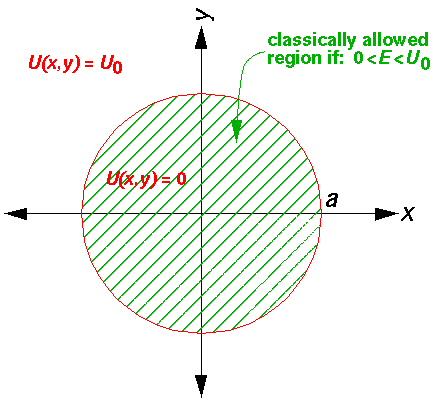In polar coordinates Schrödinger's equation reads:Inside the well, where the potential is zero, U0 would be set to zero.

Taking a as our length scale and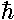2/(2ma2) as our energy scale, we gain the dimensionless formSeeking a solution where the dependence on r' factors from the dependence on("separation of variables",=R(r')()), we find: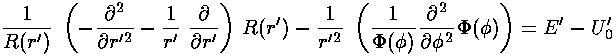The solution to theequation is easy: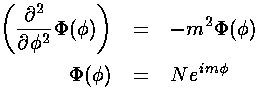Our "boundary condition" is that if we go round the origin exactly once, the value of the wavefunction is exactly the same, i.e., the wavefunction must be the same atand+2: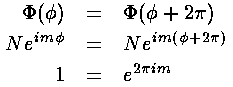Hence m must be an integer. Since the radial equation only depends on m2, the sign of m does not affect R, and we'll assume below that m is positive (i.e., in the below "m" is really |m|).

This leaves us with the r' differential equation. We will find it convenient to divide the above equation by E'-U0', but we will need to pay attention as to the sign of (E'-U0'). When (E'-U0')>0 we are in a classically allowed region, and we expect the wavefunction to oscillate with a wavelength that depends on the particle's momentum... sin(kr) like solutions are to be expected. When (E'-U0')<0 we are in a classically disallowed region, and we expect the wavefunction to exponentially decay...exp(-r) like solutions are to be expected. We'll handle both cases at the same time, sometimes by using ± signs where the top case (+ here) refers to a classically allowed region and the bottom case (- here) refers to a classically disallowed region. We substitute: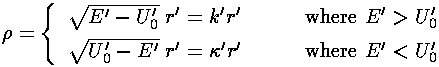in our r' differential equation, producing a differential equation which I'll write in three equivalent forms: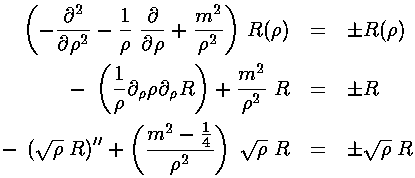As usual we'll have to work to solve the radial differential equation! Start by seeing how the the equation must work for large. If in the last equation we ignore the (very small)-2 term, we get the familiar oscillator differential equation. For E>U0 we find:That is we see the expected oscillations as a function of r', but for large r' with ever smaller amplitude, because of the 1/r½ factor.

For E<U0 we find:That is we see the exponential behavior as a function of r' expected in a classically disallowed region.

For small, the-2 term dominates and we can ignore the right hand side in comparison. If we try a power-law solutionn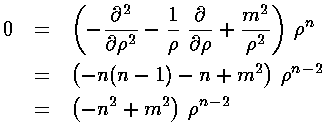we find n=|m|.

Factoring out all the required behavior for small, we hope to find a simple power series for R: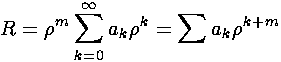Plugging this in to the first R equation: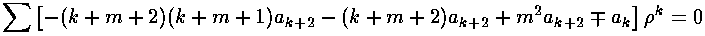Or:(In the above we've used m where we actually mean |m|.) The result is a two term recursion relation (i.e., the result has just two as so, for example, given a0 we can calculate a2, from which we can calculate a4, etc. until we're done.) Note that the the recursion relation connects even k to even k and since a0 may not be zero (as the leading termm was needed to make the smalldifferential equation work). Thus the series will have only even terms and we write our even k=2i, where i is an integer.The series does not terminate; the result (for E>U0) can be recognized as the Bessel function Jm from the hypergeometric formula (0F1).As we expect that a second order linear differential equation should have two independent solutions, we're only half way there. Jm is regular at=0, the other Bessel function: Ym (also known as Nm) explodes at=0, and hence is not part of normalizable wavefunctions. Here are the basic large and small argument behaviors of Jm and Ym.As we showed above, for largewe expect oscillations like exp[±i].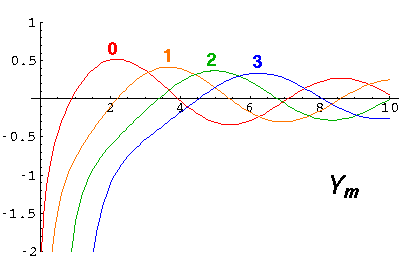Y and J are real functions and hence oscillate like sin and cos. If we want, we can put Y and J together to produce something that for largeoscillates like exp[±i]. The results are called Hankel functions: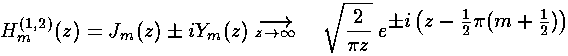For E<U0 we've found the exponentially rising modified Bessel function Im:As above there is a second solution that is exponentially falling called Km: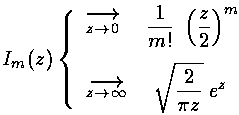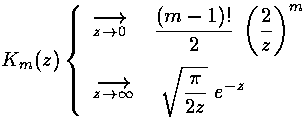### Infinite Round Square-Well

We have all our solutions, lets put them together for the simplest case: the case where U0 is infinite and so the wavefunction must be zero for r>a, i.e., r'>1. Thus we must have:

Jm(k'r')=0 for r'=1

That is k' must be a zero of Jm. The energy of the wavefunction can then be calculated from

E'=k'2

Here is the overall solution: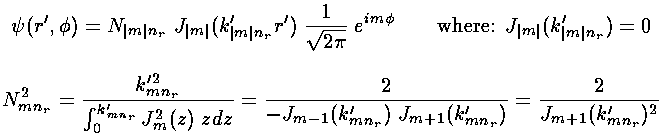Here is what the probability density looks like for nr=10, m=0 E'=1141 (again, the bright areas are places where the particle is likely to be found, the dark where it is unlikely to be found)Note the solution is circle-symmetric and has 10 nodal circles.

Here is what the probability density looks like for nr=5, m=10 E'=1038Note the solution is circle-symmetric and has 5 nodal circles, and that the particle is unlikely to be found exactly at the origin.

Of course the centrifugal potential is the source of the exclusion from the origin, but a more visual explanation comes from plotting the classical (no-force and hence straight line) trajectories of a particle with fixed angular momentum L'=10 and E'=1037.6:Note that in producing these circle-symmetric solutions, we have produced complex rather than real wavefunctions.... that factor of exp(im). This factor cancels out of our*probability density (so the probability density is circle-symmetric), but if we were to look at just the real part ofwe would find oscillation as a function of. Oscillation indicates momentum, so these solutions have angular momentum...in fact mangular momentum in the "z" direction (i.e., perpendicular to the plane).

Because the angular dependence is "simple" we can usefully plot the wavefunction just as a function of r'. Here are "stacked wavefunction" plots for m=0,1,2:The red line is the classical "effective potential": m2/r'2 (which is the "centrifugal potential") plus the infinite square well. Note that the centrifugal barrier excludes non-zero m wavefunctions from the origin.

m,nrrootE'
0,02.45.78
1,03.814.68
2,05.126.37
0,15.530.47
3,06.440.71
1,17.049.22

You should check each of the above roots on the above plots of Jm.